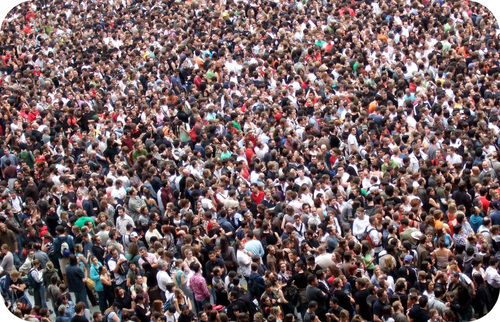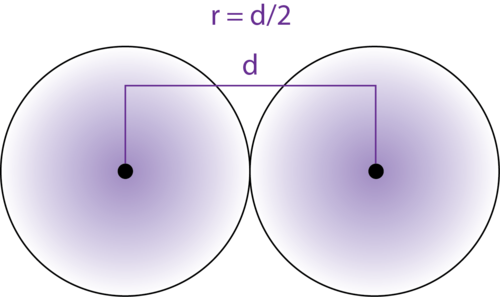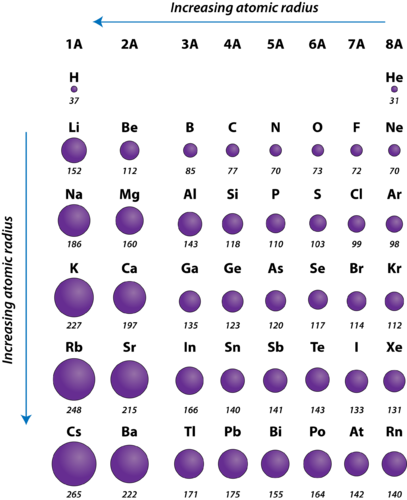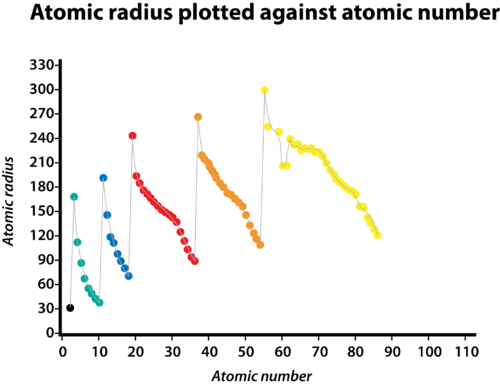### Learning Objectives

• Describe how the atomic changes within a period.
• Describe how the atomic radius changes within a group.

### How can all of these people fit in such a small space?Events draw large numbers of people to them.  Even an outdoor event can fill up so that there is no room for more people.  The crowd capacity depends on the amount of space in the venue, and the amount of space depends on the size of the objects filling it.  We can get more people into a given space than we can elephants, because the elephants are larger than people.  We can get more squirrels into that same space than we can people for the same reason.  Knowing the sizes of objects we are dealing with can be important in deciding how much space is needed.

The size of atoms is important when trying to explain the behavior of atoms or compounds.  One of the ways we can express the size of atoms is with the atomic radius .  This data helps us understand why some molecules fit together and why other molecules have parts that get too crowded under certain conditions.

The size of an atom is defined by the edge of its orbital. However, orbital boundaries are fuzzy and in fact are variable under different conditions. In order to standardize the measurement of atomic radii, the distance between the nuclei of two identical atoms bonded together is measured. The atomic radius is defined as one-half the distance between the nuclei of identical atoms that are bonded together.Figure 1. The atomic radius (r) of an atom can be defined as one half the distance (d) between two nuclei in a diatomic molecule.

Atomic radii have been measured for elements. The units for atomic radii are picometers, equal to 10−12 meters. As an example, the internuclear distance between the two hydrogen atoms in an H2 molecule is measured to be 74 pm. Therefore, the atomic radius of a hydrogen atom is $\frac{74}{2}=37\text{ pm}$.Figure 2. Atomic radii of the representative elements measured in picometers.

## Periodic Trend

The atomic radius of atoms generally decreases from left to right across a period. There are some small exceptions, such as the oxygen radius being slightly greater than the nitrogen radius. Within a period, protons are added to the nucleus as electrons are being added to the same principal energy level. These electrons are gradually pulled closer to the nucleus because of its increased positive charge. Since the force of attraction between nuclei and electrons increases, the size of the atoms decreases. The effect lessens as one moves further to the right in a period because of electron-electron repulsions that would otherwise cause the atom’s size to increase.

## Group Trend

The atomic radius of atoms generally increases from top to bottom within a group. As the atomic number increases down a group, there is again an increase in the positive nuclear charge. However, there is also an increase in the number of occupied principle energy levels. Higher principal energy levels consist of orbitals which are larger in size than the orbitals from lower energy levels. The effect of the greater number of principal energy levels outweighs the increase in nuclear charge and so atomic radius increases down a group.Figure 3. A graph of atomic radius plotted versus atomic number. Each successive period is shown in a different color. As the atomic number increases within a period, the atomic radius decreases.

### Summary

• Atomic radius is determined as the distance between the nuclei of two identical atoms bonded together.
• The atomic radius of atoms generally decreases from left to right across a period.
• The atomic radius of atoms generally increases from top to bottom within a group.

### Practice

1. What influences the atomic size of an atom?
2. What is a covalent radius?
3. What is an ionic radius?